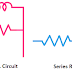The main difference between the RL Circuit, RC Circuit, and LC Circuit is the RL circuit is built with a Resistor and Inductor whereas the RC circuit is built with a resistor and capacitor, and the LC circuit is built with a resistor and inductor. The full form of the RL circuit is the Resistor-Inductor Circuit, here the resistor is denoted by R and the Inductor is denoted by L. The Full form of the RC circuit is the Resistor-Capacitor Circuit. Here the resistor is denoted by R and the Capacitor is denoted by C. On the other hand, the full form of the LC circuit is the Inductor-Capacitor Circuit. Here, the inductor is denoted by L, and the Capacitor is denoted by C. By the combination of Resistor(R), Inductor(L), and Capacitor(C) RLC circuit can be built.

## What is RL Circuit?

RL circuit is built with a resistor connecting with an inductor either in series or parallel. When the resistor is connected in series with the inductor it is called the RL Series circuit and when the resistor is connected in parallel with the inductor then it is called RL parallel circuit. RL circuit is also called an RL filter or RL network.

The RL circuit is driven by some electrical power in the form of a voltage source or current source. Now let's see what happens when we connect AC supply and Supply.

When we apply a DC supply to an RL series circuit the current flow will be high because here the inductor does not affect anymore to the DC power supply. Here, the total resistance of the circuit will be the total resistance of the resistor and the internal resistance of the inductor.

When we apply an AC supply to an RL series circuit, the current flow will be lower than when we apply a DC supply. This is because we know that the inductor blocks the AC supply due to its self-inductance property. The frequency of the power supply is also a very important factor, more frequency means more blocking by the inductor. Of course, the RL series circuit and RL parallel Circuit act differently.

## What is RC Circuit?

An RC circuit is built with a resistor connecting with a capacitor either in series or parallel. When the resistor is connected in series with the capacitor it is called an RC series circuit and when the resistor is connected in parallel with the capacitor then it is called RC parallel circuit. The RC circuit is also known as RC Filter or RC Network.

The RC circuit is also driven by an electrical power source in the form of voltage or current. It also behaves differently for different types of power sources.

For example, when we apply a DC supply to a series RC circuit the capacitor will block the current because we know that the capacitor blocks the DC current. But when we apply the AC supply the capacitor will allow the AC current. Here, also the frequency of the power source is very important. More frequency means less capacitive reactance so the current flow will be more.

## What is LC Circuit?

LC circuit is built with an inductor connecting with a capacitor either in series or in parallel. When the inductor is connected in series with the capacitor it is called an LC series circuit and when the inductor is connected in parallel with the capacitor then it is called an LC parallel circuit. The LC circuit is also known as the LC tank circuit, resonant circuit, or tuned circuit. LC series circuit can be used as a filter circuit.

The working principle of the LC circuit is quite different from the RC circuit or RL circuit. Due to the charging and discharging properties of the capacitor, the parallel LC circuit or LC tank circuit can generate an oscillating signal. LC tank circuit can be used for two main purposes either to generate an oscillating signal at a particular frequency or to extract a signal of a particular frequency from a complex signal.

## The Key Differences between RL, RC, and LC Circuit

 RL Circuit RC Circuit LC Circuit Built with Resistor and Inductor Built with Resistor and Capacitor Built with Inductor and Capacitor Basically, acts as a filter circuit Basically, acts as a filter circuit Basically, acts as a resonant circuit It causes a lagging power factor It causes a leading power factor It causes either a leading or lagging power factor It cannot generate an oscillating signal It also cannot generate an oscillating signal It can generate an oscillating signal Power dissipation is more due to the presence of a resistor Power dissipation is more due to the presence of a resistor Power dissipation is low due to the absence of a resistor

Difference between RL Circuit, RC Circuit, and LC CircuitReviewed by Author on April 23, 2022 Rating: 5